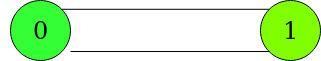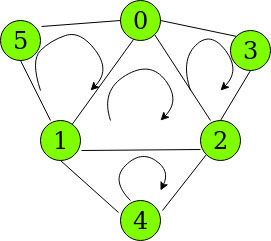Open in App
Not now

# Count of all cycles without any inner cycle in a given Graph

• Last Updated : 26 May, 2021

Given an undirected graph consisting of N vertices numbered [0, N-1] and E edges, the task is to count the number of cycles such that any subset of vertices of a cycle does not form another cycle.
Examples:

Input: N = 2, E = 2, edges = [{0, 1}, {1, 0}]
Output:
Explanation:
Only one cycle exists between the two vertices.Input: N = 6, E = 9, edges = [{0, 1}, {1, 2}, {0, 2}, {3, 0}, {3, 2}, {4, 1}, {4, 2}, {5, 1}, {5, 0}]
Output:
Explanation:
The possible cycles are shown in the diagram below:Cycles such as 5 -> 0 -> 2 -> 1 -> 5 are not considered as it comprises of inner cycles {5 -> 0 -> 1} and {0 -> 1 -> 2}

Approach:
Since V vertices require V edges to form 1 cycle, the number of required cycles can be expressed using the formula:

`(Edges - Vertices) + 1`

Illustration:

N = 6, E = 9, edges = [{0, 1}, {1, 2}, {0, 2}, {3, 0}, {3, 2}, {4, 1}, {4, 2}, {5, 1}, {5, 0}]
Number of Cycles = 9 – 6 + 1 = 4
The 4 cycles in the graph are:
{5, 0, 1}, {0, 1, 2}, {3, 0, 2} and {1, 2, 4}

This formula also covers the case when a single vertex may have a self-loop.
Below is the implementation of the above approach:

## C++

 `// C++ implementation for the``// above approach.` `#include ``using` `namespace` `std;` `// Function to return the``// count of required cycles``int` `numberOfCycles(``int` `N, ``int` `E,``                   ``int` `edges[])``{``    ``vector<``int``> graph[N];``    ``for` `(``int` `i = 0; i < E; i++) {``        ``graph[edges[i]]``            ``.push_back(edges[i]);``        ``graph[edges[i]]``            ``.push_back(edges[i]);``    ``}` `    ``// Return the number of cycles``    ``return` `(E - N) + 1;``}` `// Driver Code``int` `main()``{``    ``int` `N = 6;``    ``int` `E = 9;``    ``int` `edges[] = { { 0, 1 },``                       ``{ 1, 2 },``                       ``{ 2, 0 },``                       ``{ 5, 1 },``                       ``{ 5, 0 },``                       ``{ 3, 0 },``                       ``{ 3, 2 },``                       ``{ 4, 2 },``                       ``{ 4, 1 } };``    ``int` `k = numberOfCycles(N, E,``                           ``edges);` `    ``cout << k << endl;``    ``return` `0;``}`

## Java

 `// Java implementation for the``// above approach.``import` `java.util.*;` `class` `GFG{` `// Function to return the``// count of required cycles``static` `int` `numberOfCycles(``int` `N, ``int` `E,``                          ``int` `edges[][])``{``    ``@SuppressWarnings``(``"unchecked"``)``    ``Vector []graph = ``new` `Vector[N];``    ``for``(``int` `i = ``0``; i < N; i++)``        ``graph[i] = ``new` `Vector();``        ` `    ``for``(``int` `i = ``0``; i < E; i++)``    ``{``        ``graph[edges[i][``0``]].add(edges[i][``1``]);``        ``graph[edges[i][``1``]].add(edges[i][``0``]);``    ``}` `    ``// Return the number of cycles``    ``return` `(E - N) + ``1``;``}` `// Driver Code``public` `static` `void` `main(String[] args)``{``    ``int` `N = ``6``;``    ``int` `E = ``9``;``    ``int` `edges[][] = { { ``0``, ``1` `},``                      ``{ ``1``, ``2` `},``                      ``{ ``2``, ``0` `},``                      ``{ ``5``, ``1` `},``                      ``{ ``5``, ``0` `},``                      ``{ ``3``, ``0` `},``                      ``{ ``3``, ``2` `},``                      ``{ ``4``, ``2` `},``                      ``{ ``4``, ``1` `} };``                      ` `    ``int` `k = numberOfCycles(N, E, edges);` `    ``System.out.print(k + ``"\n"``);``}``}` `// This code is contributed by Amit Katiyar`

## Python3

 `# Python3 implementation for the``# above approach.`` ` `# Function to return the``# count of required cycles``def` `numberOfCycles(N, E, edges):` `    ``graph``=``[[] ``for` `i ``in` `range``(N)]``    ` `    ``for` `i ``in` `range``(E):``    ` `        ``graph[edges[i][``0``]].append(edges[i][``1``]);``        ``graph[edges[i][``1``]].append(edges[i][``0``]);`` ` `    ``# Return the number of cycles``    ``return` `(E ``-` `N) ``+` `1``;`` ` `# Driver Code``if` `__name__``=``=``'__main__'``:``    ` `    ``N ``=` `6``;``    ``E ``=` `9``;``    ``edges ``=` `[ [ ``0``, ``1` `],``                       ``[ ``1``, ``2` `],``                       ``[ ``2``, ``0` `],``                       ``[ ``5``, ``1` `],``                       ``[ ``5``, ``0` `],``                       ``[ ``3``, ``0` `],``                       ``[ ``3``, ``2` `],``                       ``[ ``4``, ``2` `],``                       ``[ ``4``, ``1` `] ];``    ` `    ``k ``=` `numberOfCycles(N, E,edges);``    ``print``(k)``    ` `    ``# This code is contributed by rutvik_56`

## C#

 `// C# implementation for the``// above approach.``using` `System;``using` `System.Collections.Generic;``class` `GFG{` `// Function to return the``// count of required cycles``static` `int` `numberOfCycles(``int` `N, ``int` `E,``                          ``int` `[,]edges)``{` `    ``List<``int``> []graph = ``new` `List<``int``>[N];``    ``for``(``int` `i = 0; i < N; i++)``        ``graph[i] = ``new` `List<``int``>();``        ` `    ``for``(``int` `i = 0; i < E; i++)``    ``{``        ``graph[edges[i, 0]].Add(edges[i, 1]);``        ``graph[edges[i, 1]].Add(edges[i, 0]);``    ``}` `    ``// Return the number of cycles``    ``return` `(E - N) + 1;``}` `// Driver Code``public` `static` `void` `Main(String[] args)``{``    ``int` `N = 6;``    ``int` `E = 9;``    ``int` `[,]edges = { { 0, 1 }, { 1, 2 },``                     ``{ 2, 0 }, { 5, 1 },``                     ``{ 5, 0 }, { 3, 0 },``                     ``{ 3, 2 }, { 4, 2 },``                     ``{ 4, 1 } };``                      ` `    ``int` `k = numberOfCycles(N, E, edges);` `    ``Console.Write(k + ``"\n"``);``}``}` `// This code is contributed by Rohit_ranjan`

## Javascript

 ``

Output:

`4`

Time Complexity: O(E)
Auxiliary Space: O(N)

My Personal Notes arrow_drop_up